# 杨辉三角形

``````public class YangHui {
public static void main(String[] args) {
/**
* 6行6列的杨辉三角
*/
int row = 6;//行数
int[][] yanghui = new int[row][row];//6行6列数组
for (int i = 0; i < row; i++){//行
for(int j = 0;j<= i;j++){//列
if (j==0 || j==i){
yanghui[i][j]=1;
}else{
yanghui[i][j]=yanghui[i-1][j-1]+yanghui[i-1][j];
}
System.out.print(yanghui[i][j]+" ");
}
System.out.println();
}
}
}

``````

# 求数组中的最大、最小、平均数、总和等

``````public class TestArray04 {
public static void main(String[] args) {
// 最大值
int max = 0 ;
// 最小值
int min = 0 ;
// 总和
int sum = 0 ;
// 平均值
int avg = 0 ;
int[] arrays = {1,2,3,4,66,8,9,10};
// 计算最大值
for (int i = 0 ; i < arrays.length ; i++ ){
if( max < arrays[i]){
max = arrays[i] ;
}
}
System.out.println( max );
// 计算最小值
for (int i = 0 ; i < arrays.length ; i++ ){
// 因为在上边进行min的初始化，所以这里应该将min设置为数组中的元素,可以是任意元素
min = arrays ;
if( min > arrays[i]){
min = arrays[i] ;
}
}
System.out.println( min );

// 计算总和
for (int i = 0 ; i < arrays.length ; i++ ){
sum += arrays[i] ;
}
System.out.println( sum );

// 计算平均数
System.out.println( sum / arrays.length );
}
}

``````

# 数组的复制

``````
/**
* @ClassName TestArray05
* @Description 复制数组
* @Author lujiapeng
**/
public class TestArray05 {
public static void main(String[] args) {
int[] source = {1,2,3,4,5,6,7,8} ;
// 明确数组长度
int[] dest = new int[ source.length ] ;
for (int i = 0 ; i < source.length ; i ++ ){
dest[i] = source[i] ;
}
// 循环输出 dest数组，查看是否一致
for (int j : dest) {
System.out.println( j );
}
// 使用 System 中的方法，进行复制
/**
* 方法描述 ：
*  arraycopy(Object src,  int  srcPos, Object dest, int destPos, int length);
*   src : 表示原数组
*   srcPos ： 表示 原数组 要复制的位置
*   dest ： 目标数组
*   destPos ： 目标数组 开始的下标
*   length ： 复制元素的个数（也就是要复制几个）
*  那么这个方法就可以随心的复制，同时可以明确是任意类型的数组。
*  注意：目标数组和原数组的类型要匹配
*/
System.arraycopy( source , 0 , dest , 0 , source.length );
// 循环输出 dest数组，查看是否一致
for (int j : dest) {
System.out.println( j );
}

/**
* 使用Arrays中的API，可以查看源码，底层依旧用的是 System.arraycopy
*  Arrays.copyOf 与 Arrays.copyOfRange 两种方法
*/
// 复制了 10 个元素
dest = Arrays.copyOf( source ,10  ) ;
// 循环输出 dest数组，查看是否一致
for (int j : dest) {
System.out.println( j );
}
}
}

``````

# 数组的查找

## 线性查找

``````/**
* @ClassName TestArray06
* @Description 线性查找
* @Author lujiapeng
**/
public class TestArray06 {
public static void main(String[] args) {
int[] source = {1,2,3,4,5,6,7,8} ;
int key= 8;
for(int i = 0 ; i < source.length ; i++ ){
if( source[i] == key ){
System.out.println( "下标为："+i );
break ;
}else if( (i+1) == source.length ){
System.out.println("没有找到");
}
}
}
}

``````

## 二分查找

``````/**
* @ClassName TestArray07
* @Description 二分查找
* @Author lujiapeng
**/
public class TestArray07 {
public static void main(String[] args) {
int[] source = {1,2,3,4,5,6,7,8} ;
int key= 18;
boolean isFlag = true ;
// 首索引位置
// 尾索引位置
int end = source.length - 1;
int middle = ( head + end ) / 2 ;
if( source[middle] == key ){
System.out.println("找到指定元素，索引为：" + middle );
isFlag = false ;
break ;
}else if( source[middle] > key ){
end = middle -1 ;
}else{
// 此时表示的状态就是 source[middle] < key
head = middle + 1 ;
}
}
if( isFlag ){
System.out.println("未找到指定元素");
}
}
}

``````

API实现：

``````/**
* @ClassName TestArray08
* @Description 二分查找之使用JavaAPI
* @Author lujiapeng
**/
public class TestArray08 {
public static void main(String[] args) {
int[] source = {1,2,3,4,5,6,7,8} ;
int key= 1;
/**
* 如果找到了对应的元素，则返回下标，如果没有找到，则返回一个负数。
* 如果在一个数组中有多个相同元素，则不保证返回是具体的某一个（也就是在这些元素中随机返回一个）
*/
int j = Arrays.binarySearch( source , key );
System.out.println( j );
}
}

``````

# 排序算法

## 排序的基本概念

• 排序：是计算机程序设计中的一项重要操作，其功能是指一个数据元素集合或序列重新排列成一个按数据元素某个数据项值有序的序列。
• 排序码（关键码）：排序依据的数据项
• 稳定排序：排序前与排序后相同关键码元素间的位置关系，保持一致的排序方法。
• 不稳定排序：排序前与排序后相同关键码元素间的相同位置发生改变的排序方法。

• 内排序：指待排序列完全存放在内存中所进行的排序。内排序大致可分为五类：插入排序、交换排序、选择排序、归并排序和分配排序。
• 外排序：指排序过程中还需外存储器的排序。

• 时间复杂度：分析比较的次数和记录的移动次数
• 空间复杂度：分析排序算法中许哟啊多少辅助内存
• 稳定性：若两个记录A和B的关键字值相等，但排序后A、B的先后次序保 持不变，则称这种排序算法是稳定的

## 插入排序

### 直接插入排序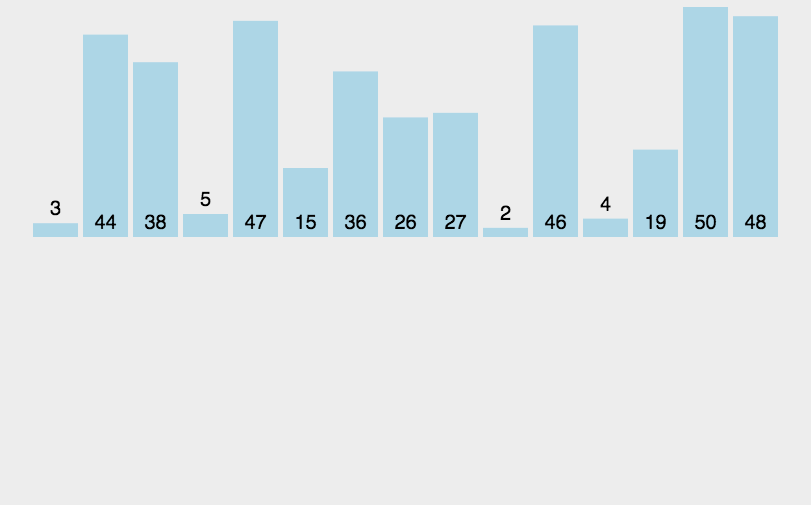``````/**
* @ClassName InsertSort
* @Description 直接插入算法
* @Author lujiapeng
**/
public class InsertSort {
public static void main(String[] args) {
int[] ints = {101, 92, 3, 34, 24, 234};
//直接插入排序
//把第一个数看成是有序的 ，所以从第二个数开始循环。
for (int i = 1; i < ints.length; i++) {
//内层循环 是从外层 循序的 前一个数开始 比
for (int j = i - 1; j >= 0; j--) {
// 如果前一个 比后一个大
if (ints[j] > ints[j + 1]) {
int temp = ints[j] ;
ints[j] = ints[j+1] ;
ints[j+1] = temp ;
}
}
}
System.out.println(Arrays.toString(ints));
}
}
``````

### 希尔排序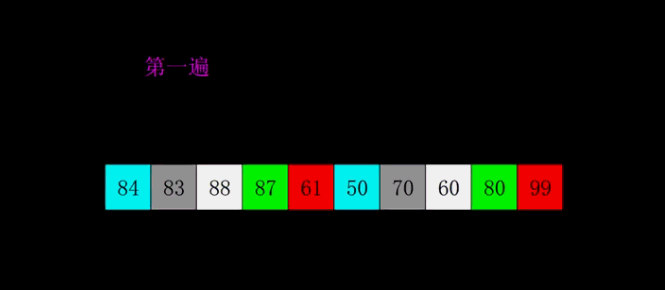``````/**
* @ClassName XierSort
* @Description TODO
* @Author lujiapeng
**/
public class XierSort {
public static void main(String[] args) {
int[] ints = {101, 92, 3, 34, 24, 234};
// 步长
for (int tap = ints.length / 2; tap > 0; tap /= 2) {
for (int i = tap; i < ints.length; i++) {
for (int j = i - tap; j >= 0; j -= tap) { // 控制每组的元素
if (ints[j] > ints[j + tap]) { // 如果逆序
int temp = ints[j]; // 则交换位置
ints[j] = ints[j + tap];
ints[j + tap] = temp;
}
}
}
}
System.out.println(Arrays.toString(ints));
}
}
``````

## 交换排序

### 冒泡排序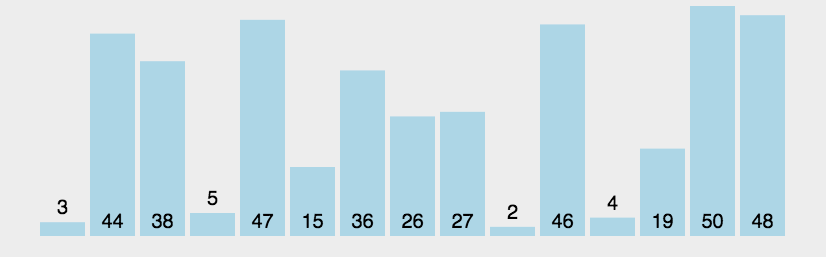``````/**
* @ClassName EffervesceSort
* @Description TODO
* @Author lujiapeng
**/
public class EffervesceSort {
public static void main(String[] args) {
//冒泡排序算法
int[] numbers = new int[]{1, 5, 8, 2, 3, 9, 4};
for ( int i = 0; i < numbers.length - 1; i++) {
for (int j = 0; j < numbers.length - 1 - i; j++) {
if (numbers[j] > numbers[j + 1]) {
int temp = numbers[j];
numbers[j] = numbers[j + 1];
numbers[j + 1] = temp;
}

}
}
System.out.println(Arrays.toString( numbers ));
}
}
``````

### 快速排序( 分区交换排序 )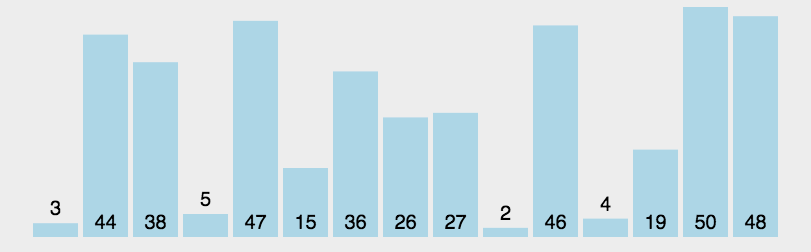``````/**
* @ClassName QuickSort
* @Description TODO
* @Author lujiapeng
**/
public class QuickSort {
public static void main(String[] args) {
int[] arr = {5, 1, 7, 3, 1, 6, 9, 4};
quickSort(arr, 0, arr.length - 1);
System.out.println(Arrays.toString( arr ));
}
/**
* @param arr        待排序列
* @param leftIndex  待排序列起始位置
* @param rightIndex 待排序列结束位置
*/
private static void quickSort(int[] arr, int leftIndex, int rightIndex) {
if (leftIndex >= rightIndex) {
return;
}
int left = leftIndex;
int right = rightIndex;
//待排序的第一个元素作为基准值
int key = arr[left];
//从左右两边交替扫描，直到left = right
while (left < right) {
while (right > left && arr[right] >= key) {
//从右往左扫描，找到第一个比基准值小的元素
right--;
}
//找到这种元素将arr[right]放入arr[left]中
arr[left] = arr[right];
while (left < right && arr[left] <= key) {
//从左往右扫描，找到第一个比基准值大的元素
left++;
}
//找到这种元素将arr[left]放入arr[right]中
arr[right] = arr[left];
}
//基准值归位
arr[left] = key;
//对基准值左边的元素进行递归排序
quickSort(arr, leftIndex, left - 1);
//对基准值右边的元素进行递归排序。
quickSort(arr, right + 1, rightIndex);
}
}
``````

## 选择排序

### 简单选择排序

1. 在以组元素R[i]到R[n] 中选择具有最小关键码的元素
2. 若它不是这组元素中的第一个元素，则将它与这组元素中的第一个元素对调。
3. 除去具有最小关键字的元素，在剩下的元素中重复1、2不，直到剩余元素只有一个为止。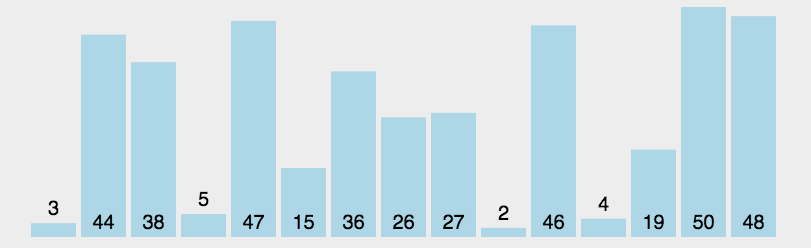``````/**
* 直接选择排序
* @author shkstart
*/
public class SelectSort {
public static void selectSort(int[] data) {
System.out.println("开始排序");
int arrayLength = data.length;
for (int i = 0; i < arrayLength - 1; i++) {
for (int j = i + 1; j < arrayLength; j++) {
if (data[i] - data[j] > 0) {
int temp = data[i];
data[i] = data[j];
data[j] = temp;
}
}
System.out.println(java.util.Arrays.toString(data));
}
}

public static void main(String[] args) {
int[] data = { 9, -16, 21, 23, -30, -49, 21, 30, 30 };
System.out.println("排序之前：\n" + java.util.Arrays.toString(data));
selectSort(data);
System.out.println("排序之后：\n" + java.util.Arrays.toString(data));
}
}

``````

## 归并排序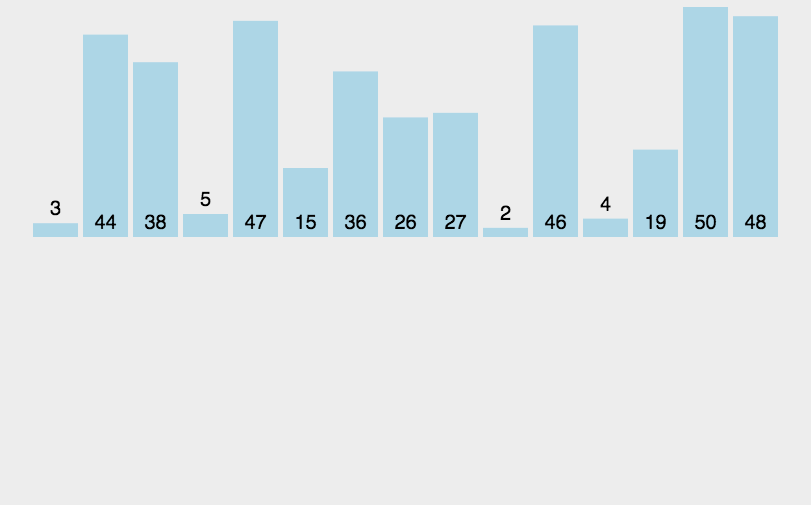``````/**
* 归并排序
*
* @author lujiapeng
*/
public class MergeSort {
public static void mergeSort(int[] data) {
// 归并排序
sort(data, 0, data.length - 1);
}

// 将索引从left到right范围的数组元素进行归并排序
private static void sort(int[] data, int left, int right) {
if(left < right){
//找出中间索引
int center = (left + right)/2;
sort(data,left,center);
sort(data,center+1,right);
//合并
merge(data,left,center,right);
}
}

// 将两个数组进行归并，归并前两个数组已经有序，归并后依然有序
private static void merge(int[] data, int left, int center, int right) {
int[] tempArr = new int[data.length];
int mid = center + 1;
int third = left;
int temp = left;
while (left <= center && mid <= right) {
if (data[left] - data[mid] <= 0) {
tempArr[third++] = data[left++];
} else {
tempArr[third++] = data[mid++];
}
}
while (mid <= right) {
tempArr[third++] = data[mid++];
}
while (left <= center) {
tempArr[third++] = data[left++];
}
while (temp <= right) {
data[temp] = tempArr[temp++];
}
}

public static void main(String[] args) {
int[] data = { 9, -16, 21, 23, -30, -49, 21, 30, 30 };
System.out.println("排序之前：\n" + java.util.Arrays.toString(data));
mergeSort(data);
System.out.println("排序之后：\n" + java.util.Arrays.toString(data));
}
}
``````### 卢佳鹏

2013/11/03
3.5K
6

2014/03/22
662
1
【opencv】图形的绘制

1.矩形图像的绘制： 原函数：void cvRectangle(CvArr* img, CvPoint pt1, CvPoint pt2, CvScalar color, int thickness=1, int line_type=8,int shift=0) img就是需要绘制的图像 pt1 and pt......

2014/10/08
1.2K
1

2013/11/09
6.6K
8

2013/11/09
4.2K
1

matplotlib基础绘图命令之imshow

0
0
[Bazel]自定义工具链

1 前言 2 Non-Platform 方式 3 Platform 方式 3.1 平台 3.2 工具链 3.3 Platform + Toolchain 实现平台方式构建 4 小结 1 前言 本文会讲述 Bazel 自定义工具链的两种方式，Platform 和 Non-...

0
0

lintao111

0
0

0
0

0 1 公司简介 字节跳动AI Lab，成立于2016年，致力于开发为字节跳动内容平台服务的创新技术，不仅仅是进行理论研究，我们的想法还可以通过实验证明和快速跟踪用于产品部署。 人工智能涉及的研...

0
0